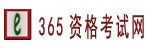首页>造价工程师>历年真题>正文
2016年造价师真题及答案《案例分析》

www.zige365.com 2017-5-12 15:07:54 点击：电子书  课件下载  考试题库 评论 收藏

某企业拟于某城市新建一个工业项目，该项目可行性研究相关基础数据如下：

1.拟建项目占地面积30亩，建筑面积11000m2，其项目设计标准，规模与该企业2年前在另一城市的同类项目相同已建同类项目的单位建筑工程费用为1600元/m2，建筑工程的综合用工量为4.5工日/m2，综合工日单价为80元/工日，建筑工程费用中的材料费占比为50%，机械使用费占比为8%，考虑地区和交易时间差拟建项目的综合工日单价为100元/工日，材料费修正系数为1:1，机械使用费的修正数为1.05，人材机以外的其它费用修正系数为1.08。

根据市场询价，该拟建项目设备投资估算为2000万元，设备安装工程费用为设备投资的15%。项目土地相关费用按20万元/亩计算，除土地外的工程建设其他费用为项目建安工程费用的15%，项目的基本预备费率为5%，不考虑价差预备费。

2.项目建设期1年，运营期10年，建设投资全部形成固定资产。固定资产使用年限为10年，残值率为5%，直线法折旧。

3.项目运营期第1年投入自有资金200万元作为运营期的流动资金。

4.项目正常年份销售收入为1560万元，营业税金及附加税率为6%，项目正常年份年经营成本为400万元。项目运营第1年产量为设计产量的85%，运营期第2年及以后各年均达到设计产量，运营期第1年的销售收入，经营成本均为正常年份的85%。企业所得税率为25%。

问题：

1.列式计算拟建项目的建设投资。

【答案】

调整前：

人工费=4.5×80=360

材料费=1600×50%=800

机械费=1600×8%=128

其它费=1600-360-800-128=312

调整后：

人工费=4.5×100=450

材料费=800×1.1=880

机械费=128×1.05=134.4

其它费=312×1.08=336.96

合计：450+880+134.4+336.96=1801.36

建筑工程费=1801.36×1.1=1981.50万元

设备费=2000万元

安装工程费=2000×15%=300万元

土地费=600万元

工程建设其它费(除土地费)=(1981.50+300)×15%=342.23万元

基本预备费=(2000+1981.50+300+600+342.23)×5%=261.19万元

建设投资=2000+1981.50+300+600+342.23+261.19=5484.92万元

【考点】建设项目投资估算与财务评价

2.若该项目的建设投资为5500万元，建设投资来源为自有资金和贷款，贷款为3000万元，贷款年利率为7.2%(按月利息)，约定的还款方式为运营期前5年等额还本，利息照付方式，分期列式计算项目运营期第1年，第2年的总成本费用和净利润以及运营期第2年年末的项目累计盈余资金，(不考虑企业公积金，公益金提取及资者股利分配)(计算结果保留两位小数)

【答案】

I=(1+7.2%/12)12-1=7.44%

Q=3000/2×7.44%=111.6万元

每年还款=(3000+111.6)/5=622.32万元

折旧=(5500+111.6)×(1-5%)/10=533.10万元

第一年利息=(3000+111.6)×7.44%=231.50万元

第二年利息=(3000+111.6-622.32)×7.44%=185.20万元

第一年总成本费用=400×85%+533.10+231.50=1104.6万元

第二年总成本费用=400+533.10+185.2=1118.3万元

第一年所得税=【1560×85%×(1-6%)-1104.6】×25%=35.46万元

第二年所得税=【1560×(1-6%)-1118.3】×25%=87.03万元

第一年净利润=1560×85%×(1-6%)-1104.6-35.46=106.38万元

第二年净利润=1560×(1-6%)-1118.3-87.03=261.07万元

第一年末盈余资金=1560×85%-(400×85%+1560×85%×6%+35.46+622.32+231.5)=17.16万元

第二年末盈余资金=1560-(400+1560×6%+87.03+622.32+185.2)=171.85万元

第二年末累计盈余资金=17.16+171.85=189.01万元

【考点】建设项目投资估算与财务评价相关新闻